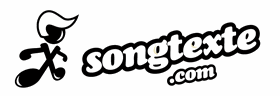# Where We Belong Songtext von Purple Disco Machine

## Where We Belong Songtext

And we finally found the place where we belong
In the darkness, we′ve been searching there for long
When we finally saw the way
Then we're gonna know
′Cause we finally found the place where we belong, right where we belong, oh yeah

(In the darkness, we've been searching there for long)
And we finally found the place where we belong
In the darkness, we've been searching there for long
When we finally saw the way
Then we′re gonna know
′Cause we finally found the place where we belong, right where we belong, oh yeah

Right where we belong
Right where we belong
Right where we belong
Right where we belong, now

Right where we belong
Right where we belong
Right where we belong
Right where we belong

Right where we belong
Right where we belong
Right where we belong
Right where we belong, now

Hey

## Songtext kommentieren

Schreibe den ersten Kommentar!

## News

### Die 10 schönsten Songs für die Vorweihnachtszeit

29. November 2021

2. Dezember 2021

2. Dezember 2021

18. März 2021

## Fan Werden

Fan von »Where We Belong« werden:
Dieser Song hat noch keine Fans.